# 标准差和方差

## 例子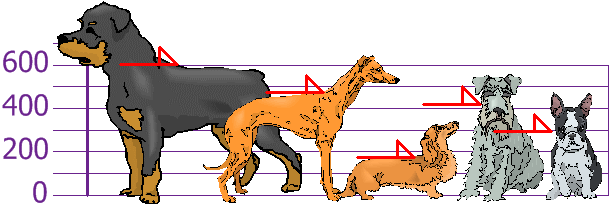### 答案：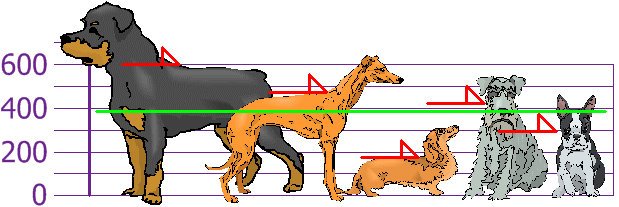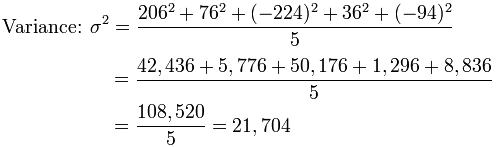标准差 σ = √21,704 = 147.32…… = 147 （到最近的毫米）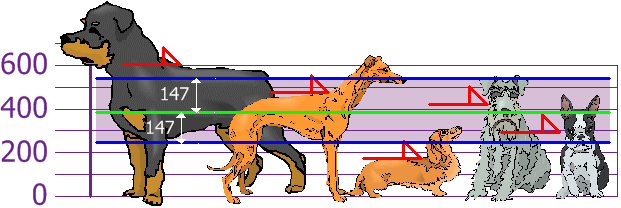## 可是……如果数据是样本数据

• 对象总体：在求方差时除以 N（如上）
• 样本：在求方差时除以 N-1

## 公式

 "对象总体标准差"：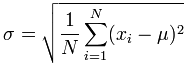"样本标准差"：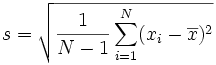### *脚注：为什么要求差的平方？4 + 4 − 4 − 44 = 0|4| + |4| + |−4| + |−4|4 = 4 + 4 + 4 + 4 4 = 4|7| + |1| + |−6| + |−2|4 = 7 + 1 + 6 + 2 4 = 4√( 42 + 42 + 42 + 424) = √( 64 4 ) = 4√( 72 + 12 + 62 + 22 4) = √( 90 4 ) = 4.74...